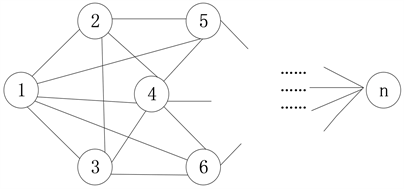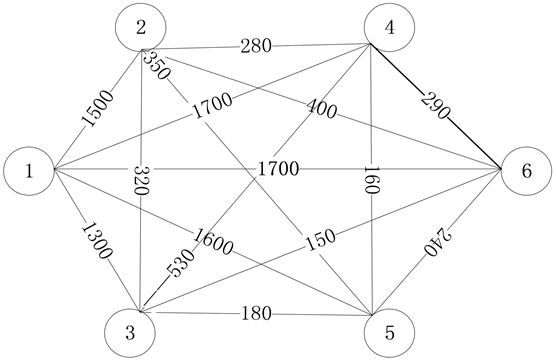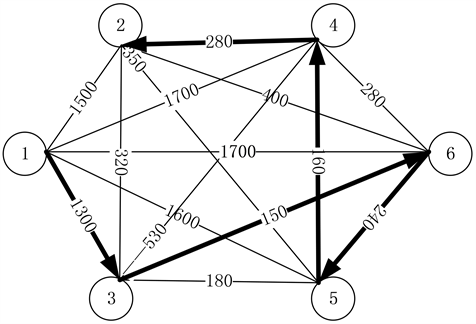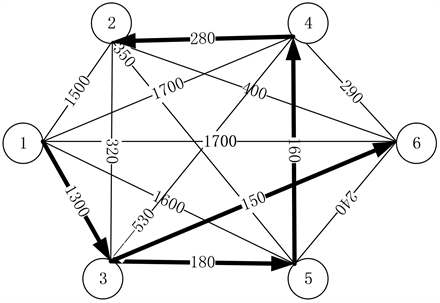# 基于Dijkstra算法的校园外卖配送方案Campus Delivery Scheme Based on Dijkstra Algorithm

• 全文下载: PDF(1040KB)    PP.38-46   DOI: 10.12677/ECL.2018.73006
• 下载量: 319  浏览量: 866

In recent years, with the development of science and technology and the improvement of people’s living standards, the emerging industry of take-out is developing rapidly, among which the fastest development is campus take-out. In this paper, by solving the problem of the shortest route of campus take-out, the problem of the delivery of campus take-out is equivalent to the shortest route problem. Dijkstra algorithm was used to obtain the best delivery scheme of campus take-out, and MATLAB program was compiled to determine the shortest route of campus take-out delivery. Finally, the validity and feasibility of the proposed algorithm are verified by an experimental example.

1. 引言

2. 模型建立

1) 区域密集性。学生宿舍一般是聚集在某个区域内，宿舍楼在区域内密集分布。

2) 时间确定性。订餐时间一般在从午九点到下午一点、下午五点到七点。

3) 路线复杂性。各宿舍楼之间的连通的路线繁复。。

1) 单条路径最短路线求解，在图1中寻找一条路径经过其中所有的连接点，使得求得最短路径的权值和最小。

2) 多条路径最短路线求解，在图1中寻找多条路径经过其中所有的连接点，使得求得最短路径的权值和最小。

2.1. 单条路径最短路线求解(附录1)

Step 1：将各点之间的权值在矩阵中表示出来， ${a}_{ij}$$i$ 点与 $j$ 点之间的权值，由此建立一个 $n×n$ 的矩阵 $A$

$A=\left(\begin{array}{cccc}{a}_{11}& {a}_{12}& \cdots & {a}_{1n}\\ {a}_{21}& {a}_{22}& \cdots & {a}_{2n}\\ \cdots & \cdots & \cdots & \cdots \\ {a}_{n1}& {a}_{n2}& \cdots & {a}_{nn}\end{array}\right)$

Step 2：比较矩阵 $A$ 的第一列，得出第一列的最小值 ${a}_{1k}$ ，即与起始点相连的点中， $k$ 点与起始点之间的权值最小。

Step 3：再以 $k$ 点为起点，找与 $k$ 点之间权值最小的点。在矩阵 $A$ 的第 $k$ 行，比较 $k$ 行中各个元素的大小，可得第 $k$ 行中最小的元素为 ${a}_{km}$ ，其含义为 $k$ 点与 $m$ 点之间的权值最小。输出点 $m$ ，将元素 ${a}_{km}$ 所在的列上所有的元素都变为无穷大值，得到矩阵 $B$

$B=\left(\begin{array}{ccccccc}{a}_{11}& \cdots & {a}_{1\left(m-1\right)}& \infty & {a}_{1\left(m+1\right)}& \cdots & {a}_{1n}\\ \cdots & \cdots & \cdots & \cdots & \cdots & \cdots & \cdots \\ {a}_{k1}& \cdots & {a}_{k\left(m-1\right)}& \infty & {a}_{k\left(m+1\right)}& \cdots & {a}_{kn}\\ \cdots & \cdots & \cdots & \cdots & \cdots & \cdots & \cdots \\ {a}_{n1}& \cdots & {a}_{n\left(m-1\right)}& \infty & {a}_{n\left(m+1\right)}& \cdots & {a}_{nn}\end{array}\right)$Figure 1. Directed graph with starting point (1) as the starting point

Step 4：以点m为起点，依照第三步的步骤进行迭代，直至路线经历所有点。根据依次输出的值的列序号就能得出单条路径情况下的最短路线。

2.2. 多条路径最短路线求解(附录2)

1) 枚举法

2) Dijkstra法

Dijkstra法是一种基于矩阵和集合的思想来求最短路径的方法，具体步骤如下：

Step 1：创立两个集合 $U,V$ ，其中 $U$ 集合存放源点，指路径出发的点， $V$ 集合存放待定点，指路径到达的终点。

Step 2：比较各源点与待定点之间的权值，将权值最小的两点连线，将此待定点加入源点集合 $U$

Step 3：重复第二步，直到待定点全部加入源点集合 $U$ 。待定点加入源点集合的顺序即路径行走的顺序。

①假设不存在孤立点，各连接点至少由一条路径与其他连接点相连；

②自身与自身相连表示权值为无穷，两点之间不相连表示权值为无穷。

$A=\left(\begin{array}{cccc}{a}_{11}& {a}_{12}& \cdots & {a}_{1n}\\ {a}_{21}& {a}_{22}& \cdots & {a}_{2n}\\ \cdots & \cdots & \cdots & \cdots \\ {a}_{n1}& {a}_{n2}& \cdots & {a}_{nn}\end{array}\right)$

①在矩阵 $A$ 中找到源点所对应的行向量 $S$ 。如以点1为源点，源点矩阵 $S=\left[{a}_{11}\text{}{a}_{12}\cdots {a}_{1n}\right]$

②在源点矩阵 $S$ 寻找权值最小的 ${a}_{ij}$ ，其中 $i$ 为源点， $j$ 为待定点。

③将 $j$ 点对应的行向量加入源点矩阵 $S$$S=\left[\begin{array}{l}{a}_{11}\text{}{a}_{12}\cdots {a}_{1n}\\ {a}_{j1}\text{}{a}_{j2}\cdots {a}_{jn}\end{array}\right]$ ，在其中以无穷大替换步骤②找出的最

2.3. 算法比较

3. 数值算例

$t=t\left(s,v\right)=\frac{s}{v}$ ，故求出最短路线的距离 $s$ 即可。

Step 1：作出路线图Table 1. The distance between any two pointsFigure 2. A road map from known data

Step 2：将表1使用矩阵的形式表述

$A=\left(\begin{array}{cccccc}0& 1500& \text{1300}& \text{1700}& \text{1600}& \text{1800}\\ 1500& 0& 320& 280& 350& 400\\ 1300& 320& 0& 530& 180& 150\\ 1700& 280& 530& 0& 160& 290\\ 1600& 350& 180& 160& 0& 240\\ 1800& 400& 150& 290& 240& 0\end{array}\right)$

$A=\left(\begin{array}{cccccc}\propto & 1500& \text{1300}& \text{1700}& \text{1600}& \text{1800}\\ 1500& \propto & 320& 280& 350& 400\\ 1300& 320& \propto & 530& 180& 150\\ 1700& 280& 530& \propto & 160& 290\\ 1600& 350& 180& 160& \propto & 240\\ 1800& 400& 150& 290& 240& \propto \end{array}\right)$

Step 3：最短路线求解

①单人配送外卖方案

$U=\left[\text{01300150240160280}\right]$$V=\left[\text{136542}\right]$$d=2130$

②多人配送外卖方案

$U=\left[\text{01300150180160280}\right]$$V=\left[\text{136542}\right]$$d=2070$Figure 3. The shortest route to deliver take-out by one personFigure 4. The shortest route to delivery take-out by many people

function [U,V,d]=lcxz(A)

b=inf;

B=A;

U=zeros(1,n);

V=ones(1,n);

R=b*ones(1,n);

L=b*ones(m,1);

a=A(1,:);

B(1,:)=R;

B(:,1)=L;

t=1;

U(1,2)=u;

V(1,2)=v;

for i=2:n-1%循环计算经过路径

s=v;

f=B(v,:);

t=i+t;

U(1,i+1)=u;

V(1,i+1)=v;

B(s,:)=R;

B(:,s)=L;

i=i+1;

end

U;%存储最短两点之间的权值

V;%存储最短路线经过的节点

d=sum(U);%最短路线的总距离

function [U,V,d]=ndjtsl(A)

b=inf;

C=inf*ones(n);

B=A;

U=zeros(1,n);

V=ones(1,n);

rep=b;

a=B(1,:);

B(1,v)=rep;

B(v,1)=rep;

U(1,2)=u;

V(1,2)=v;

f=B(1,:);

for i=3:n %循环计算经过路径

r=v;

F=[f;B(v,:)];

rv=v;

U(1,i)=u;

x=v;

s=V(x);

y=rv(v);

v=y;

V(1,i)=y;

B(r,y)=rep;

B(y,r)=rep;

B(s,y)=rep;

B(y,s)=rep;

f=[f;B(r,:)];

f(x,y)=rep;

i=i+1;

end

U;%存储最短两点之间的权值

V;%存储最短路线经过的节点

d=sum(U);%最短路线的总距离

  邓佩, 苏翔. 时间约束下的运输网络最短路径研究[J]. 机电产品开发与创新, 2006, 19(1): 18-20.  王树西, 李安渝. Dijkstra算法中的多邻接点与多条最短路径问题[J]. 计算机科学, 2014, 41(6): 217-224.  周康, 同小军, 刘文斌, 等. 最短路问题的闭环DNA算法[J]. 系统工程与电子技术, 2008, 30(3): 556-560.  赵礼峰, 梁娟. 最短路问题的Floyd改进算法[J]. 计算机技术与发展, 2014(8): 31-34.  冯树民, 吴海月, 王弟鑫. 基于理想点法的多目标最短路求解算法研究[J]. 公路交通科技, 2016, 33(3): 97-101.  丁秋雷, 胡祥培, 姜洋. 基于前景理论的物流配送干扰管理模型研究[J]. 管理科学学报, 2014, 17(11): 1-9.